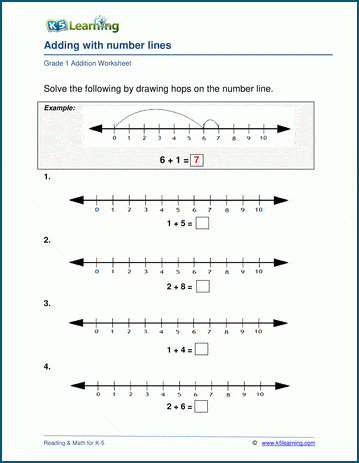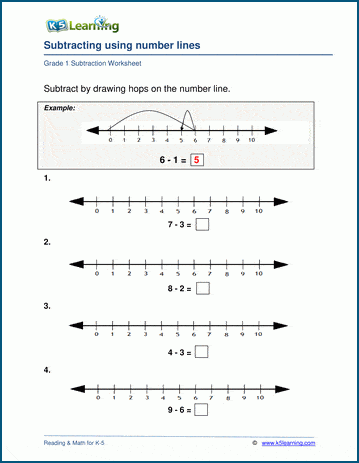# Addition and Subtraction on a Number Line

## What is the number line?

A number line is a line on which numbers are marked at intervals, be they whole numbers, fractions or integers. It is often used to illustrate simple numerical operations, such as addition, subtraction and multiplication.

## Why do we use number lines in math learning?

Number lines add a visual element to students learning numerical concepts, especially for young students who are learning to add and subtract simple numbers.  This method of teaching is important because, students are able to visualize the operations and, therefore, able to learn and understand these basic math operations quickly.

## Video on using number lines for adding and subtracting

We have created a 60 second video to demonstrate how single-digit addition and subtraction is completed on a number line to help students quickly grasp simple addition and subtraction.

For students who want some practice at how to use the number lines for addition and subtraction, we have created worksheets for just that.